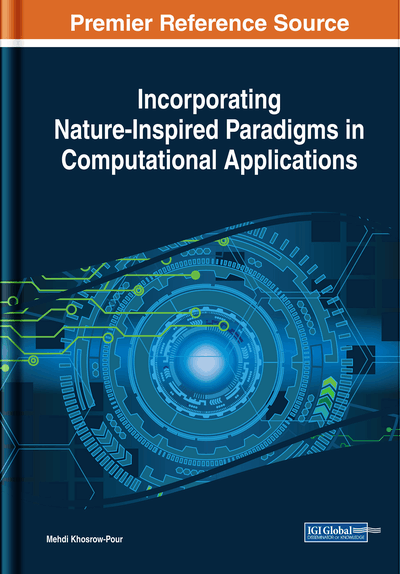# Application of Natural-Inspired Paradigms on System Identification: Exploring the Multivariable Linear Time Variant Case

Mateus Giesbrecht, Celso Pascoli Bottura
DOI: 10.4018/978-1-5225-5020-4.ch001
OnDemand:
(Individual Chapters)
Available
\$29.50
No Current Special Offers

## Abstract

In this chapter, the application of nature-inspired paradigms on system identification is discussed. A review of the recent applications of techniques such as genetic algorithms, genetic programming, immuno-inspired algorithms, and particle swarm optimization to the system identification is presented, discussing the application to linear, nonlinear, time invariant, time variant, monovariable, and multivariable cases. Then the application of an immuno-inspired algorithm to solve the linear time variant multivariable system identification problem is detailed with examples and comparisons to other methods. Finally, the future directions of the application of nature-inspired paradigms to the system identification problem are discussed, followed by the chapter conclusions.
Chapter Preview
Top

## Introduction

For more than 50 years, many techniques have been developed to control a huge variety of dynamic systems, which vary from simple and well-known mechanical systems to the dynamics of the financial market. To develop those control techniques, it is fundamental to know the mathematical relations between the system inputs and outputs. This necessity naturally led to the development of techniques to find the mathematical realizations of the systems to be controlled. These realizations are mathematical models that, for a given domain, behave as close as possible to the system to be modeled. The determination of the realizations of a given dynamical system is defined as system identification.

Given the huge variety of the existent systems, there are many models that can be used to generate a system realization and, for this reason, there are also many techniques that can be applied to reach this objective. Generally, the starting point is the information available about the system to be studied. Depending on the available information, one of those three techniques may be applied: the white, grey or black box modelling. Those techniques might be applied to any kind of system, including linear, non-linear, time variant, time invariant, monovariable and multivariable ones.

If the laws that govern the system behavior are reasonably known and if there is an estimate of the parameters involved in the problem, the system equations can be written from the known dynamics using the estimated parameters and a mathematical realization is then determined. This technique is known as white-box identification, since all the relevant details about the system are available. The models obtained with this technique are reasonably accurate for simple and well-studied systems operating on a known region of the domain.

For more complex physical systems, sometimes the structure of the equations is known but the parameters are not, either because they are complicated to measure or because their values vary depending on the ambient conditions. To obtain realizations for those systems, it is possible to write the equations that relate their outputs to their inputs as functions of the unknown parameters, to collect input and output data from the system operation and to estimate the parameters as the set that minimizes the error between the real system outputs and the model outputs. It is also possible to collect the output signal statistical characteristics and to determine the set of parameters for which the model produces the outputs with the maximum likelihood to the real system outputs. Those methods are known respectively as error minimization and maximum likelihood. In this case, where the model structure is known but the parameters are not, the system identification using any of the methods cited is known as grey-box.

If both the system structure and the parameters are unknown, there is necessary to apply techniques capable to determine the model structure and the parameters. There is also important to establish an information criterion to reach a compromise between the model accuracy and the model complexity, in order to avoid too complex models that do not result in an accuracy much better than simpler ones. For linear systems, the subspace methods allow the determination of generic state space models without the necessity of any initial guess about the system order. For non-linear systems, linear combinations of the results of non-linear functions applied to the inputs can be used to model the unknown dynamics. These nonlinear functions must be bases of the functions space, such as polynomials, sigmoid funcions, radial basis functions, etc. That functions can also be applied recursively, as observed on neural networks. Once a technique is defined, the identification procedure consists on defining the set of parameters and model structures that minimize the information criterion. In this case, the identification method is known as black-box, since no information about the system is available a priori.

For the grey-box and the black-box approaches, the system identification is an optimization problem, where the objective is to find the solutions that minimize the output error, or maximize the likelihood between the systems and the model outputs, or minimize the information criterion. From this point of view, natural inspired paradigms might be used to solve this kind of problem, since many of those paradigms were developed to determine the solutions of optimization problems, such as the evolutionary algorithms.

For the time variant system identification, the application of evolutionary algorithms is even more attractive, since in this case the system parameters vary along the time, and the evolutionary approach allows the population of possible solutions to continuously evolve to the optimal set of parameters at each time instant.

## Complete Chapter List

Search this Book:
Reset